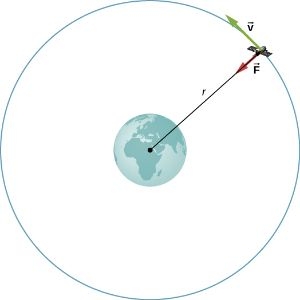# A satellite orbits the moon at height of 2000 m. Assuming it to the gravitational pull of the...

## Question:

A satellite orbits the moon at height of 2000 m. Assuming it to the gravitational pull of the moon, find the speed and time it takes for one orbit, given that mass of moon is {eq}7.34 \times 10^{22}\; kg {/eq} and radius is {eq}1.738\times10^6\;m {/eq}.(g = {eq}6.6\times 10^{-11}\;Nm^2/kg {/eq}).

## Satellite of Satellite:

Whether an artificial satellite rotates around a planet or a natural satellite, it follows same laws.Let an artificial satellite be rotating round a planet of mass {eq}M {/eq} and radius {eq}R {/eq} at a height {eq}h {/eq} from the surface of the planet.

Gravitational Constant is {eq}G {/eq}.

Then angular speed of the satellite can be given by:

{eq}\displaystyle{\omega = \sqrt{\frac{GM}{(R + h)^{3}}}} {/eq}.

Time period of revolution of the satellite can be given by:

{eq}\displaystyle{T = 2\pi\sqrt{\frac{(R + h)^{3}}{GM}}} {/eq}.

Speed of the satellite can be given by:

{eq}\displaystyle{v = \sqrt{\frac{GM}{R + h}}} {/eq}.

Mass of the moon: {eq}\color{blue}{M = 7.34\times 10^{22}\, \rm kg} {/eq}.

Radius of the moon: {eq}\color{blue}{R = 1.738\times 10^{6}\, \rm m} {/eq}.

Height of the satellite orbiting the moon: {eq}\color{blue}{h = 2000\, \rm m} {/eq}.

Gravitational constant: {eq}\color{blue}{G = 6.6\times 10^{-11}\, \rm \frac{Nm^{2}}{kg^{2}}} {/eq}.

Speed of the satellite can be given by:

{eq}\displaystyle{\begin{align*} \color{red}{v} &= \color{blue}{\sqrt{\frac{GM}{R + h}}}\\ &= \color{blue}{\sqrt{\frac{6.6\times 10^{-11}\times 7.34\times 10^{22}}{1.738\times 10^{6} + 2000}}}\\ &\approx \color{red}{1,668.57\, \rm m/s}\\ \end{align*}} {/eq}

Time period of revolution of the satellite, i.e. time it takes to complete one revolution is given by:

{eq}\displaystyle{\begin{align*} \color{red}{T} &= \color{blue}{2\pi\sqrt{\frac{(R + h)^{3}}{GM}}}\\ &= \color{blue}{2\pi\sqrt{\frac{(1.738\times 10^{6} + 2000)^{3}}{6.6\times 10^{-11}\times 7.34\times 10^{22}}}}\\ &\approx \color{red}{6552.15\, \rm s}\\ \end{align*}} {/eq}